# SPC/HW model of water

Jump to: navigation, search

The SPC/HW model is an SPC model designed for heavy water. The molecule is modelled as a rigid isosceles triangle, having charges situated on each of the three atoms. Apart from Coulombic interactions, the molecules interact via long-range Lennard-Jones sites, situated on the oxygen atoms, i.e. (Eq. 1 in ):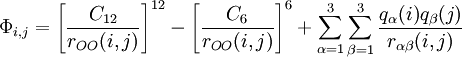$\Phi_{i,j}= \left[ \frac{C_{12}}{r_{OO}(i,j)}\right]^{12} - \left[ \frac{C_{6}}{r_{OO}(i,j)}\right]^{6} + \sum_{\alpha=1}^3 \sum_{\beta=1}^3 \frac{q_\alpha(i) q_\beta(j)}{r_{\alpha \beta}(i,j)}$

The parameters are as follows (Ref 1 Table I. Note, in the table the HOH (DOD) angle is given as 109.43 but in the text as 109.47):

 parameter value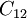$C_{12}$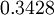$0.3428$ (kJ mol-1) 1/12nm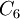$C_6$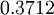$0.3712$ (kJ mol-1) 1/6nm$r_\mathrm{OH}$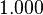$1.000$ Å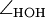$\angle_\mathrm{HOH}$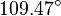$109.47^{\circ}$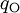$q_{\mathrm{O}}$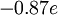$-0.87 e$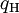$q_{\mathrm{H}}$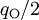$q_{\mathrm{O}}/2$ (charge neutrality)